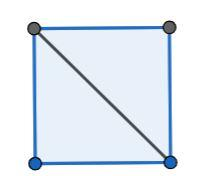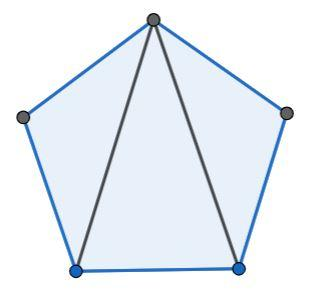QUESTION

# Express in radians and also in degrees the angle of a regular polygon of (i). 40 sides (ii). n sides.

Hint: Try to express the square and regular pentagon in terms of triangles and hence, try to impose the same for a regular polygon with sides 40 and n. Then, find the angle for them in degrees and convert it to radians by using the conversion factor $\dfrac{\pi }{{180^\circ }}$.

A polygon is a closed figure formed with straight lines.
A regular polygon is a polygon having all of its sides equal and all the angles equal.
The simplest polygon is the triangle and the sum of its angles is 180°.
A four-sided regular polygon is called a square. A square can be expressed as two triangles as shown in the figure. Hence, the sum of the angles of the square is $2 \times 180^\circ$, which is $360^\circ$.A five-sided regular polygon is called a regular pentagon. A regular pentagon can be expressed as three triangles as shown in the figure. Hence, the sum of the angles is $3 \times 180^\circ$,which is $540^\circ$.Hence, the sum of angles of a regular polygon of 40 sides is $38 \times 180^\circ$, which is $6840^\circ$.
Now, each angle is the sum of the total angles divided by the number of sides.
$\dfrac{{6840^\circ }}{{40}} = 171^\circ$
Hence, the angle of the regular polygon with 40 sides is 171°.
The sum of angles of an n-sided regular polygon is $(n - 2) \times 180^\circ$.
The value of each angle is $(n - 2) \times 180^\circ$ divided by n, which is $\dfrac{{(n - 2)}}{n} \times 180^\circ$.
To convert from degree to radians, we need to multiply the angle with $\dfrac{\pi }{{180^\circ }}$.
Hence, the angle of a 40 sided polygon is given as follows:
$171^\circ \times \dfrac{\pi }{{180^\circ }} = 0.95\pi rad$
Hence, the angle of an n- sided polygon is given as follows:
$\dfrac{{n - 2}}{n} \times 180^\circ \times \dfrac{\pi }{{180^\circ }} = \dfrac{{n - 2}}{n}\pi rad$
Hence, we found the required values.

Note: You can also directly use the formula for the value of the angle of an n-sided polygon which is $\dfrac{{(n - 2)}}{n} \times 180^\circ$ and use it to find the answers.To convert degree into radians we have to just multiply $\dfrac{\pi }{{180^\circ }}$ and to convert radians into degree we have to multiply $\dfrac{180^\circ }{{\pi }}$.